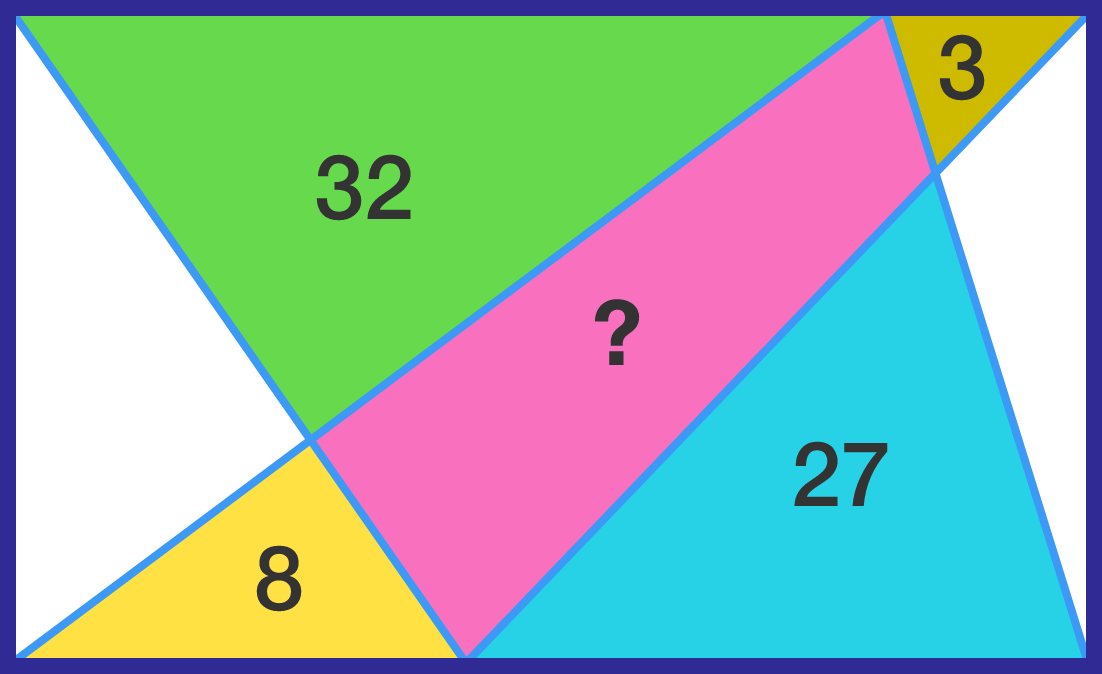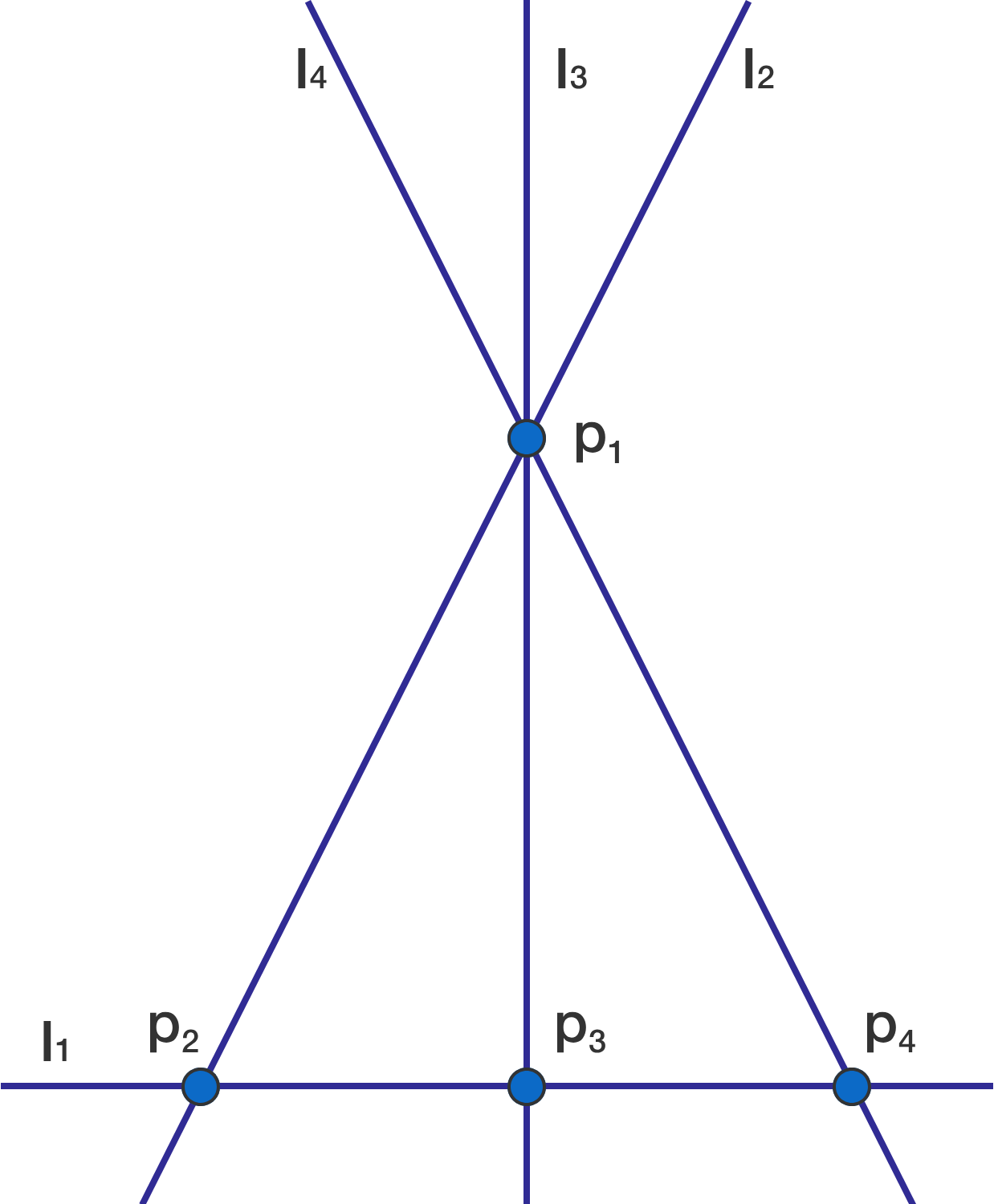# Problems of the Week

Contribute a problemA rectangle is divided by four lines, as shown, with 4 of the resulting triangles having known areas. Find the area of the quadrilateral that is shaded in pink.

$\begin{cases} a^{ 2 }+b^{ 2 }+c^{ 2 }+d^{ 2 }=1 \\\\ K=ab+ac+ad+bc+bd+3cd \end{cases}$

Let 4 real numbers $a$, $b$, $c$, and $d$ satisfy the two equations above. If the minimum and maximum values of $K$ are $m$ and $M,$ respectively, what is the value of $m+M ?$

A $k$-regular graph satisfies the neighborhood diversity condition if it is possible to label each vertex with one of $1, 2, 3, \ldots, k$ such that for each vertex, all its neighbors have different labels.

Determine the sum of all $n$ satisfying $1 \le n \le 1000$ such that the $n$-hypercube graph satisfies the neighborhood diversity condition.


Clarification:

• In a $k$-regular graph, each vertex has $k$ neighbors.
• An $n$-hypercube graph is the graph of an $n$-dimensional hypercube. In other words, its vertices are elements of $\{0,1\}^n$ (that is, $n$-tuples with each component 0 or 1), and two vertices are adjacent if and only if they differ in exactly one component.
• The labeling is not necessarily a proper vertex coloring: two adjacent vertices may have the same label.

$a^n-b^{n+2}=(a+b)^{n-1}$

$a$ and $b$ are relatively prime positive integers and $n\, (>1)$ is an integer. Find all solutions to the equation above and enter your answer as $\sum (a+b+n).$

This is a generalization of the problem from 1997 Russian Olympiad:

For prime numbers $p$ and $q,$ solve $p^3-q^5=(p+q)^2.$

On a projective plane, there are points and lines. Points may or may not lie on lines. Given any two points on the plane, there exists a unique line passing through them. Any two lines intersect at exactly one point; that is, for any two lines on the plane, there is a unique point that lie on both of them.

Suppose $P$ is a set of points and $L$ is a set of lines on a projective plane. A pair $(p, l)$ with $p \in P, l \in L$ is called an incidence if $p$ lies on $l$. Let $I(n)$ be the maximum number of incidences among all $P, L$ such that $|P| = |L| = n$.

For example, $I(4) = 9$, achieved by having $P = \{p_1, p_2, p_3, p_4\}$, $L = \{l_1, l_2, l_3, l_4\}$, and having the set of incidences

$\big\{(p_2, l_1), (p_3, l_1), (p_4, l_1), (p_1, l_2), (p_2, l_2), (p_1, l_3), (p_3, l_3), (p_1, l_4), (p_4, l_4)\big\}.$

It can be proven that no configuration can reach more than 9 incidences. Geometrically, the above example can be represented in the following diagram:However, remember that despite the objects being called points and lines, there is no requirement that they can actually be represented on the standard Euclidean plane.

As it turns out, $I(n) = \Theta(n^c)$ for some exponent $c$; in other words, there exist constants $c, N, C_1, C_2 > 0$ such that for all $n > N$, we have $C_1 \cdot n^c \le I(n) \le C_2 \cdot n^c$.

What is the value of $\lfloor 10000c \rfloor?$


Notation: $\lfloor \cdot \rfloor$ denotes the floor function.

×